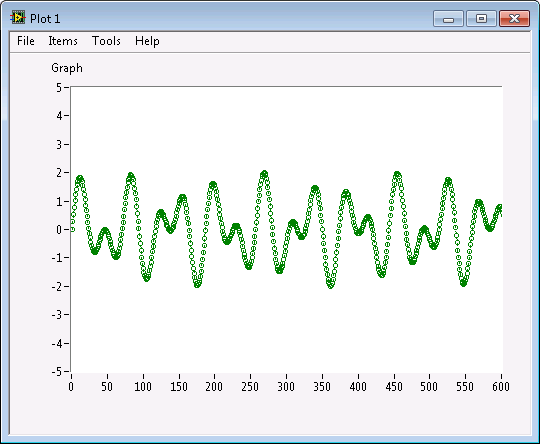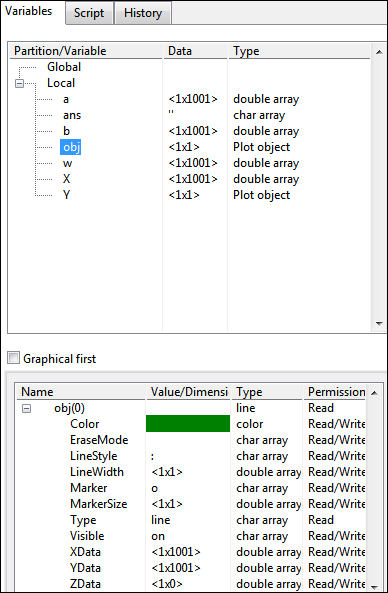# Customizing Plots in LabVIEW MathScript (MathScript RT Module)

LabVIEW 2012 MathScript RT Module Help

Edition Date: June 2012

Part Number: 373123C-01

»View Product InfoDownload Help (Windows Only)

Use the MathScript plots functions in the LabVIEW MathScript Window or the MathScript Node to create 2D and 3D plots.Note  When you create plots in a MathScript Node, you can pass the plot into the LabVIEW environment, which allows you to operate on the plot at run time.

You can customize plots by manipulating plot attributes, which are the characteristics of the different plot components. MathScript allows you to customize line attributes, plot area attributes, plot window attributes, and text attributes.

To customize plots using plot attributes, choose from the following methods:

• Specifying plot attribute values when you create a plot
• Using functions that operate on plot objects
• Modifying plot attributes in the LabVIEW MathScript Window through the user interface

You can create the following plot with each of these three methods.## Method 1: Specifying Plot Attribute Values when You Create a Plot

If a function in the plots class accepts attributes, you can specify the attributes in the script. You can create Plot 1 with the following script:

w = 0:0.1:100;

a = sin(w);

b = sin(1.7 * w);

X = a + b;

plot(X, 'Color', 'g', 'Marker', 'o', 'Linestyle', ':')

Y = axes;

set(Y, 'YLim', [-5, 5], 'XLim', [0, 600]);

As shown in the previous script, the plot function generates the 2D plot and plot(X, 'Color', 'g', 'Marker', 'o', 'Linestyle', ':') sets the attributes for Plot 1. You also can list the plot attributes in order, according to the attributes parameter on the help page for the function, and safely omit the attribute names, so plot(X, 'go:') also sets the attributes for Plot 1.

## Method 2: Using Functions that Operate on Plot Objects

The plot function, used in the previous script, can return a reference to the line object in the form of the obj output. You can use the obj reference and the set function to customize attribute values for the line object. If you want to create a .m file to define attribute values, you can apply customized values by calling the .m file in the script. This method allows you to apply custom attributes to many, different plots. The following example shows you how to define a custom .m file that sets the line attributes for Plot 1:

function myplots(plot_object)

set(plot_object, 'Color', 'g', 'Marker', 'o', 'Linestyle', ':');

You can call myplots.m to create Plot 1 with the following script:

w = 0:0.1:100;

a = sin(w);

b = sin(1.7 * w);

X = a + b;

obj = plot(X);

myplots(obj);

Y = axes;

set(Y, 'YLim', [-5, 5], 'XLim', [0, 600]);

## Method 3: Modifying Plot Attributes in the LabVIEW MathScript Window through the User Interface

If you generate a plot using a function that creates and returns a plot object, you can modify plot attributes in the LabVIEW MathScript Window through the user interface.

The following illustration shows where you can highlight the plot object in the Variable List and change the plot attributes in the Preview Pane at the bottom of the LabVIEW MathScript Window.Refer to the MathScript Customized Plot.vi in the labview\examples\MathScript directory for an example of customizing plots.

Not Helpful